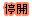課程資訊
 課程名稱 大氣動力學一Atmospheric Dynamics (Ⅰ)(Ⅰ) 開課學期 107-1 授課對象 理學院  大氣科學研究所 授課教師 課號 AtmSci5065 課程識別碼 229 U5630 班次 學分 3.0 全/半年 半年 必/選修 選修 上課時間 星期一2(9:10~10:00)星期四1,2(8:10~10:00) 上課地點 備註 上課教室:大氣B105。限大氣系碩士班乙組，選課前請先與授課老師詳談?限碩士班以上 且 限本系所學生(含輔系、雙修生)總人數上限：5人 Ceiba 課程網頁 http://ceiba.ntu.edu.tw/1071AtmSci5065_ 課程簡介影片 核心能力關聯 核心能力與課程規劃關聯圖 課程大綱 為確保您我的權利,請尊重智慧財產權及不得非法影印 課程概述 本課程係以理學院，大氣科學系大三學生為授課對象，於每週一上午九時至十時，每週四上午八時至十時上課，為必修課程。學程一年，上下學期各為三學分課程。 　　使用教材為Holton, J. R., 1992: An Introduction to Dynamic Meteorology. 3rd. Ed., Academic Press, 511 pp.課程內容主要為： 0. Overview 1. Introduction - The atmospheric continuum, physical 　　dimensions, and units, scale analysis, the fundamental 　　forces, noninertial reference frames and apparent forces, 　　structure of the static atmosphere. 2. The basic conservation laws - Total differential, the 　　vertical form of the momentum equation in rotating 　　coordinates, the component equations in spherical 　　coordinates, scale analysis of the equation of motion, 　　the continuity equation, the thermodynamic energy 　　equation, thermodynamicsof the dry atmosphere. 3. Elementary application of the basic equations - The 　　basic equation in isobaric coordinates, balanced flow, 　　trajectories and streamlines, the thermal wind, vertical 　　motion, surface pressure tendency. 4. Circulation and vorticity - The circulation theorem, 　　vorticity, potential vorticity, the vorticity equation, 　　the barotropic (Rossby) potential vorticity equation, 　　the baroclinic (Ertel) potential vorticity equation. 5. The planetary boundary layer - Atmospheric turbulence, 　　turbulent kinetic energy, planetary boundary layer 　　momentum equations, secondary circulations and spin-down. 6. Synoptic-scale motions I: Quasi-geostrophic analysis - The 　　observed tructure of extratropical circulation, the quasi- 　　geostropic approxi-mation, quasi-geostropic prediction, 　　diagnosis of vertical motion, idealized model of a 　　baroclinic disturbance. 課程目標 課程開始時，先簡介大氣動力學的綱要，進而教授大氣之基本概念-單位，因次化，尺度分析及標準大氣之架構。繼之介紹基本守恆定律，大氣之基本方程及應用，環流、渦度之概念以及邊界層之理論；並將所學之概念應用於綜觀尺度天氣 課程要求 待補 預期每週課後學習時數 Office Hours 參考書目 待補 指定閱讀 待補 評量方式(僅供參考)
 課程進度
 週次 日期 單元主題